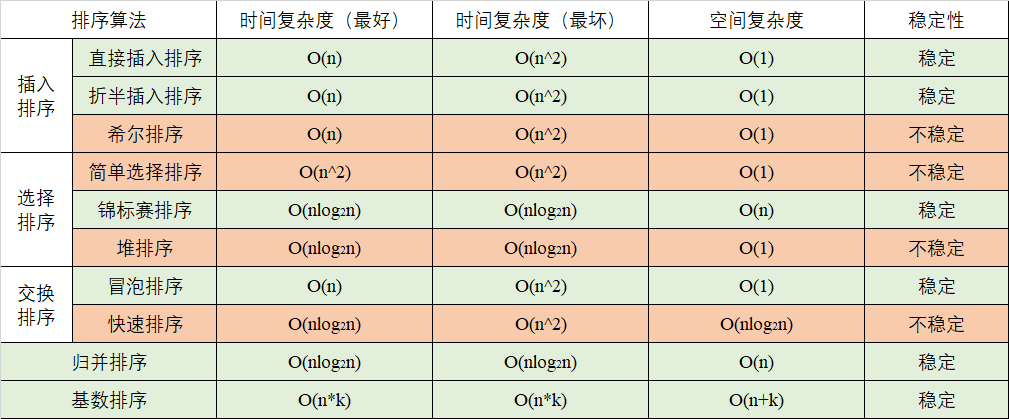# 常见内部排序算法对比分析及C++ 实现代码## 1. 直接插入排序

```void DirectInsertSort(vector<int>& arr)
{
int i, j;
for (i = 1; i < arr.size(); i++)
{
if (arr[i] < arr[i - 1])    //判断是否需要重新排序，否则保留原位置
{
int temp = arr[i];      //待插入元素
for (j = i - 1; j >= 0 && temp < arr[j]; j--) arr[j + 1] = arr[j];    //逆序寻找插入位置，同时将大于待插入元素右移
arr[j + 1] = temp;
}
}
}```

## 2. 折半插入排序

(0)
(0)